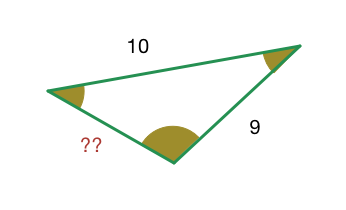# Trigonometry! #96

Geometry Level 4In a triangle, the lengths of the two larger sides are $10$ and $9$ respectively. If the angles are in A.P., then the length of the third side can be

1. $5-\sqrt{6}$

2. $3\sqrt{3}$

3. $5$

4. $5+\sqrt{6}$

Note: Enter the sum of the serial numbers of the correct options. More than one option is correct.

This problem is part of the set Trigonometry.

×